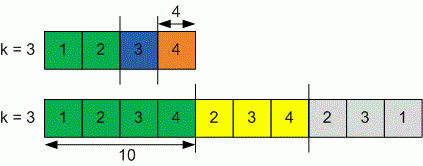favorite We need a little bit of your help to keep things running, click on this banner to learn more
Competitions

# Split into k sub-arrays

Given array of n elements and number k. Split the given array into k sub-arrays (they must cover all the elements). The maximum sub-array sum achievable out of k sub-arrays formed, must be minimum possible. Find that possible sub-array sum.

#### Input

First line contains two numbers n and k (n106, 1kn). Second line contains n positive integers, each no more than 109.

#### Output

Print the minimum possible sub-array sum.#### Explanation

For the first sample the optimal split is {1, 2}, {3}, {4}. Maximum sum of all sub-arrays is 4, which is minimum possible for 3 subarrays.

For the second sample the optimal split is {1, 2, 3, 4}, {2, 3, 4}, {2, 3, 1}. Maximum sum of all sub-arrays is 10, which is minimum possible for 3 subarrays.

Time limit 1 second
Memory limit 128 MiB
Input example #1
4 3
1 2 3 4

Output example #1
4

Input example #2
10 3
1 2 3 4 2 3 4 2 3 1
Output example #2
10